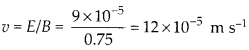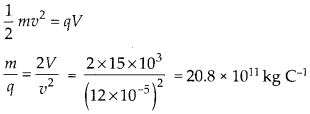Enlightened

# Question 20: NCERT Solutions for 12th Class Physics: Chapter 4-Moving Charges and Magnetism

• 0

Question 20: NCERT Solutions for 12th Class Physics: Chapter 4-Moving Charges and Magnetism

A magnetic field set up using Helmholtz coils (described in question 16 above) is uniform in a small region and has a magnitude of 0.75 T. In the same region, a uniform electrostatic field is maintained in a direction normal to the common axis of the coils. A narrow beam of (single species) charged particles all accelerated through 15 kV enters this region in a direction perpendicular to both the axis of the coils and the electrostatic field. If the beam remains undeflected when the electrostatic field is 9.0 × 10-5 V m-1, make a simple guess as to what the beam contains. Why is the answer not unique?

Share

1. Solution:
Narrow beam of charged particles remains undeflected and is perpendicular to both electric field and magnetic fields which are mutually perpendicular. So, the electric force is balanced by magnetic force. qE = quB Speed of charged particlesBecause the beam is accelerated through 15 kV, if charge is q, then kinetic energy gained by charged particlesHere, we can only obtain charge to mass ratio and same ratio can be in Deuterium ions, He++, Li+++, so the beam can contain any of these charged particles.

Check the complete chapter with solutions.

NCERT Solutions for 12th Class Physics: Chapter 4-Moving Charges and Magnetism

• 0